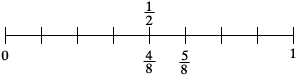SEARCH HOMEMath Central Quandaries & QueriesQuestion from jessie, a student: I'm having alot of trouble with ordering fractions from least to greatest! I'm in 5th grade....and my problem is, 5/8,1/2, and 3/4 I need to know HOW to order them.........can anyone give me a simple explanation?Hi Jessie,

To order fractions you need to express them with the same denominator. Your three fractions can all be expressed with a denominator of 8. 5/8 already has a denominator of 8. 1/2 = (1 × 4)/(2 × 4) = 4/8 and 4/8 is less than 5/8 so you know that 1/2 is less than 5/8. Now find an equivalent fraction to 3/4 with a denominator of 8. Where does it fit?PennyMath Central is supported by the University of Regina and The Pacific Institute for the Mathematical Sciences.